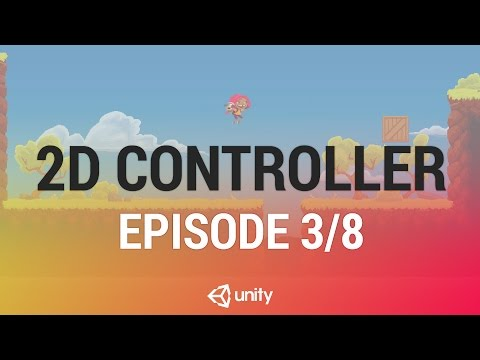# Detecting Overlaps

#### 난이도: 중급

In this live training session we will learn how to create a character controller for a 2D platform game which uses custom physics instead of Unity’s built in 2D Physics.## Detecting Overlaps

중급 2D Game Creation

## PhysicsObject

### Code snippet

``````using System.Collections;
using System.Collections.Generic;
using UnityEngine;

public class PhysicsObject : MonoBehaviour {

public float minGroundNormalY = .65f;
public float gravityModifier = 1f;

protected Vector2 targetVelocity;
protected bool grounded;
protected Vector2 groundNormal;
protected Rigidbody2D rb2d;
protected Vector2 velocity;
protected ContactFilter2D contactFilter;
protected RaycastHit2D[] hitBuffer = new RaycastHit2D;
protected List<RaycastHit2D> hitBufferList = new List<RaycastHit2D> (16);

protected const float minMoveDistance = 0.001f;
protected const float shellRadius = 0.01f;

void OnEnable()
{
rb2d = GetComponent<Rigidbody2D> ();
}

void Start ()
{
contactFilter.useTriggers = false;
}

void Update ()
{
targetVelocity = Vector2.zero;
ComputeVelocity ();
}

protected virtual void ComputeVelocity()
{

}

void FixedUpdate()
{
velocity += gravityModifier * Physics2D.gravity * Time.deltaTime;
velocity.x = targetVelocity.x;

grounded = false;

Vector2 deltaPosition = velocity * Time.deltaTime;

Vector2 moveAlongGround = new Vector2 (groundNormal.y, -groundNormal.x);

Vector2 move = moveAlongGround * deltaPosition.x;

Movement (move, false);

move = Vector2.up * deltaPosition.y;

Movement (move, true);
}

void Movement(Vector2 move, bool yMovement)
{
float distance = move.magnitude;

if (distance > minMoveDistance)
{
int count = rb2d.Cast (move, contactFilter, hitBuffer, distance + shellRadius);
hitBufferList.Clear ();
for (int i = 0; i < count; i++) {
}

for (int i = 0; i < hitBufferList.Count; i++)
{
Vector2 currentNormal = hitBufferList [i].normal;
if (currentNormal.y > minGroundNormalY)
{
grounded = true;
if (yMovement)
{
groundNormal = currentNormal;
currentNormal.x = 0;
}
}

float projection = Vector2.Dot (velocity, currentNormal);
if (projection < 0)
{
velocity = velocity - projection * currentNormal;
}

float modifiedDistance = hitBufferList [i].distance - shellRadius;
distance = modifiedDistance < distance ? modifiedDistance : distance;
}

}

rb2d.position = rb2d.position + move.normalized * distance;
}

}``````

## PlayerPlatformerController

### Code snippet

``````using System.Collections;
using System.Collections.Generic;
using UnityEngine;

public class PlayerPlatformerController : PhysicsObject {

public float maxSpeed = 7;
public float jumpTakeOffSpeed = 7;

private SpriteRenderer spriteRenderer;
private Animator animator;

// Use this for initialization
void Awake ()
{
spriteRenderer = GetComponent<SpriteRenderer> ();
animator = GetComponent<Animator> ();
}

protected override void ComputeVelocity()
{
Vector2 move = Vector2.zero;

move.x = Input.GetAxis ("Horizontal");

if (Input.GetButtonDown ("Jump") && grounded) {
velocity.y = jumpTakeOffSpeed;
} else if (Input.GetButtonUp ("Jump"))
{
if (velocity.y > 0) {
velocity.y = velocity.y * 0.5f;
}
}

bool flipSprite = (spriteRenderer.flipX ? (move.x > 0.01f) : (move.x < 0.01f));
if (flipSprite)
{
spriteRenderer.flipX = !spriteRenderer.flipX;
}

animator.SetBool ("grounded", grounded);
animator.SetFloat ("velocityX", Mathf.Abs (velocity.x) / maxSpeed);

targetVelocity = move * maxSpeed;
}
}``````